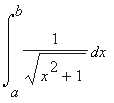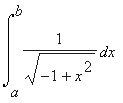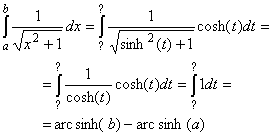#Interactive Real Analysis

Next | Previous | Glossary | Map

## 7.2. Integration Techniques

### Example 7.2.4(e): Applying the Substitution Rule

FindandNow it gets interesting again. In a previous example we had the square root of r2 - x2, and we used the substitution x = sin(t) because of the equality sin2(t) + cos2(t) = 1.

For the first integral we use the substitution

x = sinh(t) [ = 1/2 (et - e-t) ] so that
dx/dt = 1/2 (et + e-t) = cosh(t) or dx = cosh(t) dt
because of the hyperbolic trig equation
cosh2(t) - sinh2(t) = 1
(which you should verify). With that substitution we have that:where arc sinh is the inverse function of sinh. Note that it is not hard to show thatEvaluating the second integral is left as an exercise (try the substitution x = cosh(t)).
Next | Previous | Glossary | Map